• In similarity searching, there is often a query record that is compared against a stored database of records (documents or images etc). The main aim is to retrieve a set of database records that are similar to the query record.
• Similarity search works with semantic representations of data and finds the similar items fast. This means when we represent images or pieces of text as vector embeddings, their semantic similarity is represented by how close their vectors are in the vector space. Hence, what we want to look at is the distance between vectors of the objects.
• The following article has been written by Arpita Vats.

### Applications

• Image retrieval, document retrieval, recommender systems, image recognition, similar movies/items etc.
• Recently, vector databases have surged in prominence in this era of generative AI. Vector databases index the data with embedding representations of that data. They are a special type of database used for handling vector data, typically in the context of machine learning, particularly for high-dimensional data. Implementing a vector database requires understanding of several fundamental concepts:
• Vector Indexing: High-dimensional vector data cannot be effectively handled using traditional B-tree or Hash-based index structures. Instead, specialized index structures like KD-trees, Ball Trees, HNSW (Hierarchical Navigable Small World), or IVF (Inverted File) are used. These allow efficient nearest neighbor search in high dimensional spaces.
• Data Partitioning: For scalability, the data must be partitioned across multiple nodes or disks. This can be done in several ways, such as random partitioning, k-means based partitioning, or graph-based partitioning. The goal is to keep similar vectors on the same node to minimize network communication during a query.
• Query Execution: Query execution involves finding the most similar vectors to a given query vector. This can be done either exactly, which can be computationally expensive, or approximately, which is faster but may not return the exact nearest neighbors. The algorithm used depends on the index structure. For instance, with HNSW, the algorithm starts with a random node and progressively navigates to closer and closer nodes until it cannot find a closer one.
• Distributed Systems: To handle large amounts of data, vector databases must be designed as distributed systems. This involves issues like data replication for fault-tolerance, distributed query execution, and load balancing.
• Hardware Acceleration: Vector databases often utilize hardware acceleration to speed up computation. This might involve the use of GPUs for performing the vector computations, or even specialized hardware like TPUs or FPGAs.
• These are the general principles of how a vector database might be implemented. However, the specifics would depend on the use case, the available resources, and the particular trade-offs between precision, speed, and scalability that are acceptable. Popular vector databases like FAISS from Facebook AI, Spotify’s Annoy, or Google’s ScaNN (Scalable Nearest Neighbors) employ these principles in different ways to optimize for their specific needs.

## Approximate Nearest Neighbors: Overview

• Approximate Nearest Neighbor (ANN) algorithms are a type of algorithm used in the field of computer science to find the nearest neighbors to a query point in a dataset.
• As the name suggests, these algorithms are approximate, and they make a trade-off between accuracy and speed.

## Approximate Nearest Neighbors: Implementation

• Implementing an Approximate Nearest Neighbor algorithm involves a few general steps, although there are many different specific algorithms, each with its own way of implementing these steps.

High-dimensional vector data cannot be effectively handled using traditional B-tree or Hash-based index structures. Instead, specialized index structures such as K-D trees, Ball trees, Locality-Sensitive Hashing (LSH), Hierarchical Navigable Small World (HNSW), etc.

• Here is a general idea of the steps involved:
1. Preprocessing or Indexing: This is the step where the data is initially processed in some way to make the search for nearest neighbors more efficient. This often involves creating some kind of index. The way this is done depends on the algorithm. For example, in the case of k-d trees, the data is divided into a binary tree where each node is a point, and points are divided based on whether they are greater or less than the parent node in one dimension. In the case of LSH, the data is hashed in such a way that similar items are likely to be hashed to the same or nearby buckets.
2. Search: This is the step where the actual nearest neighbors are found. Again, the specifics depend on the algorithm. In general, the idea is to reduce the search space as much as possible. For example, in a k-d tree, you would start at the root and traverse down the tree, at each step going down the branch that is closer to the query point, until you reach a leaf. Then you backtrack, checking the other branch only if it could possibly contain a closer point. This process is repeated until all possible closer points are checked. In the case of LSH, you would look at the bucket that the query point hashes to, as well as some nearby buckets, to find the closest points.
3. Postprocessing: Sometimes, additional steps are necessary after the search to refine the results. For example, you might want to rerank the top k results using a more precise (but slower) distance measure.
• Each of these steps can be quite complex and involve a lot of tricky details and optimizations. The goal is to minimize the amount of data that needs to be looked at (and hence the time taken), while still getting results that are as accurate as possible. As such, many different strategies have been developed, each with its own trade-offs and best use cases.

### K-D trees

• A k-d tree, or k-dimensional tree, is a binary search tree where data in each node is a k-dimensional point in space. In other words, instead of sorting the data in the nodes along one dimension, it sorts them along $$k$$ dimensions. It is a data structure useful for applications that involve multi-dimensional keys like computational geometry and database applications.
• The primary use of k-d trees is for performing efficient range searches and nearest neighbor searches. Here’s how it works for nearest neighbor search:
1. Building the tree: The tree is built in a way where every node splits the input data in half. At each level of the tree, points are divided into two groups: those with a dimension value less than a certain point (forming the left subtree) and those with a dimension value greater or equal to it (forming the right subtree). The dimension chosen to split on can vary depending on the strategy. It may alternate between all available dimensions or choose the dimension with the greatest variance.
2. Searching for the nearest neighbor: Starting at the root, the algorithm moves down the tree recursively, in the same way as for a binary search. At each node, it first checks the subtree that the query point falls in. The reason for this is that the closest point is most likely to be in this subtree.
3. Backtracking: After the search reaches a leaf node, it backtracks, checking the other subtree if it could contain a closer point. This is determined by checking if the distance from the query point to the splitting plane at the node is less than the distance from the query point to the current closest point. If it is, that means there could be a closer point on the other side and it needs to be checked.
4. Termination: The algorithm continues this until it has ascended back up to the root of the tree, at which point all possible subtrees that could contain a closer point have been checked. The closest point found in this process is then returned as the nearest neighbor.
• K-d trees can be very efficient for nearest neighbor search, especially in low-dimensional spaces. The time complexity of the search operation in a balanced k-d tree is O(log n), where n is the number of points. However, the efficiency decreases as the number of dimensions increases, due to the “curse of dimensionality”, which makes it harder to partition the points effectively. For high-dimensional data, methods like Locality-Sensitive Hashing (LSH) or other types of space partitioning trees, like ball trees or R-trees, may be more efficient.

### Locality-Sensitive Hashing (LSH)

• Locality-Sensitive Hashing (LSH) is a method used in computer science to identify items that are close in a high-dimensional space. It is widely used for nearest neighbor search problems, especially in the field of machine learning and data mining.
• The idea of LSH is to hash input items in such a way that similar items are likely to be hashed into the same bucket. This is in contrast to most conventional hashing techniques, where the aim is to distribute items uniformly across all buckets even if they are similar.
• When applied to the problem of nearest neighbor search, the LSH method can significantly reduce the amount of data that needs to be checked. Instead of comparing the query point to every other point in the dataset, you only need to compare it to points that are in the same bucket or nearby buckets.
• Here is a simplified step-by-step implementation of an LSH-based approximate nearest neighbor search:
1. Hashing: First, you define a set of hash functions. These hash functions are designed such that the probability of collision is higher for objects that are closer to each other in the input space. Each data point is hashed using these functions, resulting in multiple hash values per point.
2. Bucketing: Each unique hash value represents a bucket. All the data points that have the same hash value (under a particular hash function) are placed in the same bucket.
3. Querying: When a query point comes in, it is hashed using the same set of hash functions. The corresponding buckets for each hash are retrieved.
4. Candidate Selection: The points in these retrieved buckets are candidate points. These are the points that are potentially close to the query point.
5. Post-Processing: The exact distance between the query point and each of the candidate points is computed and the points are ranked accordingly.
• The main challenge in LSH is designing a good set of hash functions for a particular problem. This usually requires some knowledge about the nature of the data and the definition of similarity or distance for the problem at hand.
• One common example of LSH is using random hyperplanes in a vector space. In this case, the hash function is defined by a randomly chosen hyperplane, and the hash value is determined by which side of the hyperplane a point falls on. This technique is used, for example, in cosine similarity LSH.
• The trade-off in LSH is between speed and accuracy. You can get faster results by using more hash functions and larger buckets, but this may lead to less accurate results. On the other hand, using fewer hash functions and smaller buckets can give more accurate results, but at the cost of slower search times.
• Let’s delve a bit deeper into the trade-offs in Locality-Sensitive Hashing (LSH).
• The fundamental idea behind LSH is that it uses a collection of hash functions to partition the data points into various buckets, so that similar points are more likely to fall into the same or nearby buckets. This allows us to limit the search for the nearest neighbor to just the points in the same or nearby buckets as the query point, instead of having to search the entire dataset.
• However, there’s a balancing act involved in the configuration of the hash functions and the bucket size:
1. Number of Hash Functions: More hash functions lead to a higher dimensionality of the hash space, thus allowing for a more precise partitioning of the data points. This makes the search faster, as each individual bucket is smaller, reducing the number of points that need to be compared to the query point. However, it also increases the chances of false negatives – points that are close to the query point but end up in a different bucket due to the high granularity of the partitioning. So, while the search is faster, it may also be less accurate.
2. Bucket Size: Large buckets will contain more points, meaning that a query will require checking more points for similarity, slowing down the search time. On the other hand, larger buckets reduce the chance of missing a close point (false negatives) because they are more likely to contain points that are close to the query point. In contrast, smaller buckets will contain fewer points, speeding up the search time but possibly increasing the chance of missing a close point, which would decrease accuracy.
• In summary, using more hash functions and larger buckets can speed up the search, but at the cost of possibly missing some close points, thereby reducing accuracy. Conversely, using fewer hash functions and smaller buckets can improve accuracy, but the search might be slower because each query requires checking more points. Hence, there’s a trade-off between speed and accuracy in LSH, and the optimal configuration depends on the specific requirements of your application.

### Hierarchical Navigable Small World (HNSW) graphs

• Hierarchical Navigable Small World (HNSW) is a graph-based method for performing efficient Approximate Nearest Neighbor (ANN) search in high-dimensional spaces. The method was proposed by by Malkov and Yashunin (2016) in Efficient and robust approximate nearest neighbor search using Hierarchical Navigable Small World graphs and is often used when dealing with high-dimensional data.
• HNSW is based on the concept of constructing a hierarchical graph where each node represents a point in the dataset. The graph is built in a way that allows for efficient navigation through the data to find approximate nearest neighbors. The key idea is to maintain connections between nodes in such a way that the graph exhibits small-world properties, meaning that even in high-dimensional spaces, the average distance between nodes remains relatively small.
• The HNSW algorithm addresses the “curse of dimensionality” problem encountered in many other nearest neighbor search algorithms, like k-d trees. It can handle many more dimensions and larger datasets compared to these methods.
• The idea behind HNSW is to build a hierarchy of graphs, with each graph representing the data at a different “level” of granularity. Here is a high-level overview of how it works:
1. Building the graph: HNSW starts by building a navigable small-world graph. Each point is a node in the graph and is connected to other nodes in such a way that there is a path between any two nodes that approximates the shortest path in the original high-dimensional space. The graph is constructed such that for each node, there is a balance between “local” connections (to nearby nodes) and “long-range” connections (to far-away nodes). The local connections ensure that the search can be refined, while the long-range connections ensure that the search can quickly get close to the target.
2. Creating the hierarchy: The hierarchy is created by selecting a subset of nodes to form a new graph at a higher level. Each higher level contains fewer nodes, with the highest level containing just a few nodes. The selection process is random, with each node having a certain probability of being included in the higher level.
3. Searching for the nearest neighbor: The search starts at a node at the highest level and moves to the node that is closest to the query point. This process continues until it cannot find a closer node at the current level. Then it moves down to the next lower level and continues the search. This process is repeated until it reaches the lowest level.
• This approach provides a very efficient way to find the nearest neighbors. The hierarchical structure allows the search to quickly navigate to the vicinity of the target, while the small-world property of the graphs ensures that the search can be completed in a small number of steps.
• The construction of the HNSW graph involves some tuning parameters, like the maximum number of connections per node, which can affect the trade-off between accuracy and speed. However, once the graph is built, the search process is very fast, making HNSW a popular choice for nearest neighbor search in high-dimensional spaces.
• For more, see the HNSW paper on Paper’s List.

### Visual summary

• Credits for this section go to Damien Benveniste.
• Hierarchical Navigable Small World (HNSW) graphs are one of the most efficient ways to build indexes for vector databases. The idea is to build a similarity graph and traverse that graph to find the nodes that are the closest to a query vector.
• Navigable Small World (NSW) networks is a process to build efficient graphs for search. Let’s imagine we have multiple vectors we need to index. We build a graph by adding them one after the others and connecting each new node to the most similar neighbors.
• When building the graph, we need to decide on a metric for similarity such that the search is optimized for the specific metric used to query items. Initially, when adding nodes, the density is low and the edges will tend to capture nodes that are far apart in similarity. Little by little, the density increases and the edges start to be shorter and shorter. As a consequence the graph is composed of long edges that allow us to traverse long distances in the graph, and short edges that capture closer neighbors. Because of it, we can quickly traverse the graph from one side to the other and look for nodes at a specific location in the vector space.
• When we want to find the nearest neighbor to a query vector, we initiate the search by starting at one node (i.e. node $$A$$ in that case). Among its neighbors $$(D, G, C)$$, we look for the closest node to the query ($$D$$). We iterate over that process until there are no closer neighbors to the query. Once we cannot move anymore, we found a close neighbor to the query. The search is approximate and the found node may not be the closest as the algorithm may be stuck in a local minima.
• The problem with NSW, is we spend a lot of iterations traversing the graph to arrive at the right node. The idea for Hierarchical Navigable Small World is to build multiple graph layers where each layer is less dense compared to the next. Each layer represents the same vector space, but not all vectors are added to the graph. Basically, we include a node in the graph at layer $$L$$ with a probability $$P(L)$$. We include all the nodes in the final layer (if we have $$N$$ layers, we have $$P(N) = 1$$) and the probability gets smaller as we get toward the first layers. We have a higher chance of including a node in the following layer and we have $$P(L) < P(L + 1)$$.
• The first layer allows us to traverse longer distances at each iteration where in the last layer, each iteration will tend to capture shorter distances. When we search for a node, we start first in layer 1 and go to the next layer if the NSW algorithm finds the closest neighbor in that layer. This allows us to find the approximate nearest neighbor in less iterations in average.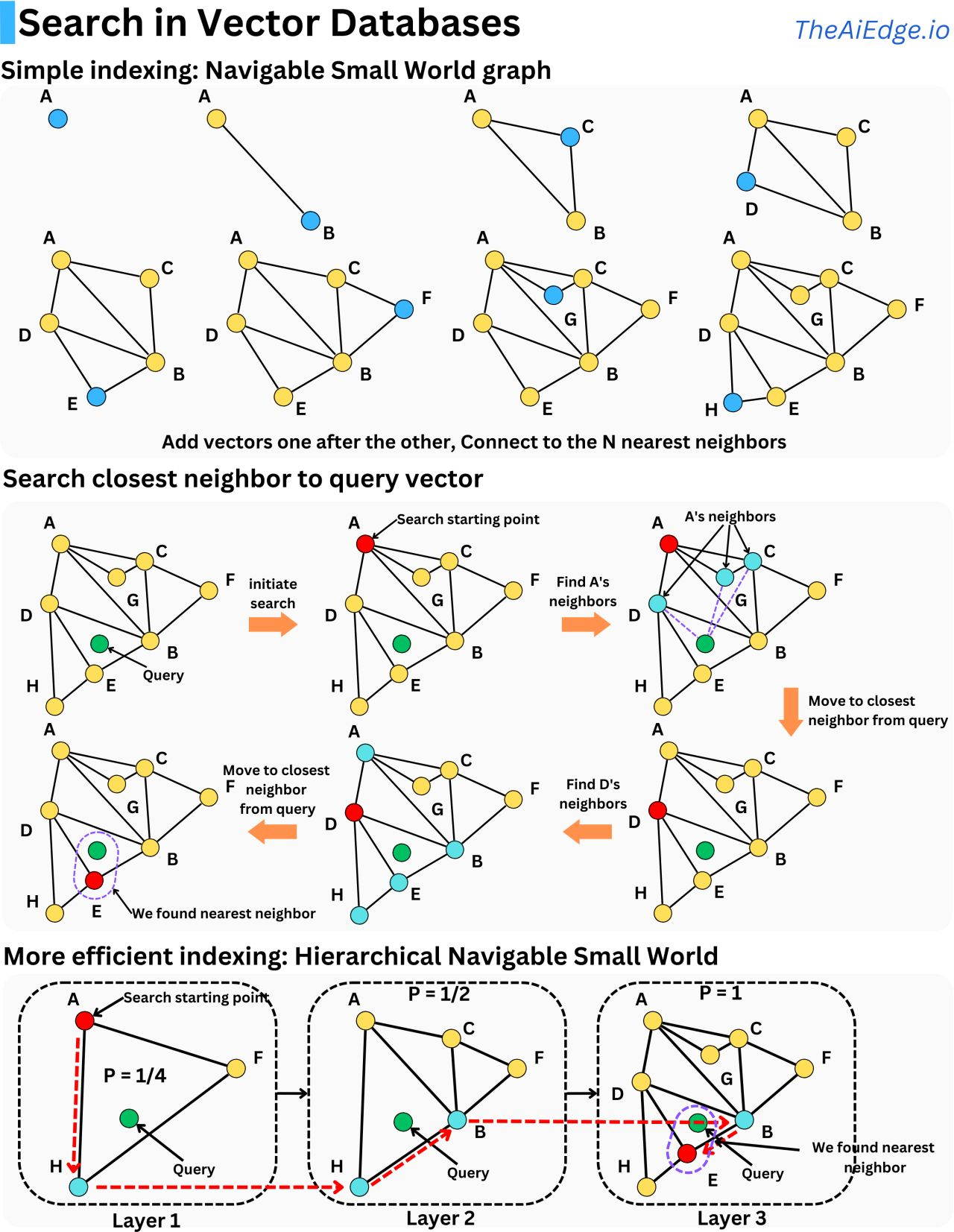## Approximate Nearest Neighbors

• Approximate Nearest Neighbors (ANN)-based search techniques like Hierarchical Navigable Small Worlds (HNSW) are commonly deployed to carry out similarity search.

## FAISS

• Facebook AI Similarity Search (FAISS) is a library developed by Facebook AI that enables efficient similarity search.
• Given a set of vectors, we can index them using FAISS then using another vector (the query vector), we search for the most similar vectors within the index. FAISS not only allows us to build an index and search but it also speeds up search times to ludicrous performance levels. FAISS provides several similar search methods that span a broad spectrum of usage trade-offs.
• FAISS is optimized for memory usage and speed.
• FAISS offers a state-of-the-art GPU implementation for the most relevant indexing methods.
• To know more about the different and efficient indexing done by FAISS, please refer Efficient Indexing of Billion-Scale datasets of deep descriptors by Babenko and Lempitsky (2016).
• The figure below shows the internal mechanism of FAISS.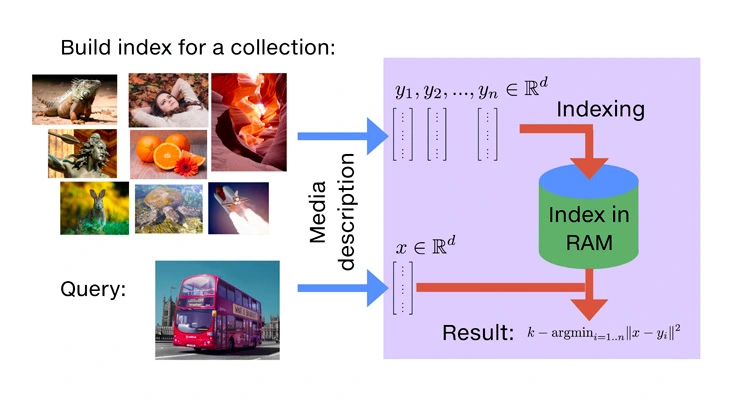• Once the vectors are extracted by learning machinery (from images, videos, text documents, and elsewhere), they’re ready to feed into the similarity search library.
• Similarity search can be made orders of magnitude faster if we’re willing to trade some accuracy; that is, deviate a bit from the reference result. For example, it may not matter much if the first and second results of an image similarity search are swapped, since they’re probably both correct results for a given query. Accelerating the search involves some pre-processing of the data set, an operation that we call indexing.
• This brings us to the three metrics of interest:
• Speed: How long does it take to find the 10 (or some other number) most similar vectors to the query? Hopefully less time than the brute-force algorithm needs; otherwise, what’s the point of indexing?
• Memory usage: How much RAM does the method require? More or less than the original vectors? FAISS supports searching only from RAM, as disk databases are orders of magnitude slower. Yes, even with SSDs.
• Accuracy: How well does the returned list of results match the brute-force search results? Accuracy can be evaluated by counting the number of queries for which the true nearest neighbor is returned first in the result list (a measure called 1-recall@1), or by measuring the average fraction of 10 nearest neighbors that are returned in the 10 first results (the “10-intersection” measure).

### Pros

• FAISS is a library for efficient similarity search and clustering of dense vectors. It contains algorithms that search in sets of vectors of any size, up to ones that possibly do not fit in RAM. It also contains supporting code for evaluation and parameter tuning.
• FAISS optimizes through various indexing techniques.

### Cons

• Similarity search can be made orders of magnitude faster if we’re willing to trade some accuracy; that is, deviate a bit from the reference result.

## ScaNN

• ScaNN (Scalable Nearest Neighbors), by Google, is a method for efficient vector similarity search at scale.
• Modern ML models can transform inputs such as text and images into embeddings, high dimensional vectors trained such that more similar inputs cluster closer together.
• For a given query, we can therefore compute its embedding, and find the literary works whose embeddings are closest to the query’s. computational challenge remains: for a given query embedding, how does one quickly find the nearest dataset embeddings? The set of embeddings is often too large for exhaustive search and its high dimensionality makes pruning difficult.
• Embedding-based search is a technique that is effective at answering queries that rely on semantic understanding rather than simple indexable properties.
• In this technique, machine learning models are trained to map the queries and database items to a common vector embedding space, such that the distance between embeddings carries semantic meaning, i.e., similar items are closer together.
• To answer a query with this approach, the system must first map the query to the embedding space. It then must find, among all database embeddings, the ones closest to the query; this is the nearest neighbor search problem.
• One of the most common ways to define the query-database embedding similarity is by their inner product; this type of nearest neighbor search is known as maximum inner-product search (MIPS).
• Referring to the figure below source: Announcing ScaNN: Efficient Vector Similarity Search, the two-tower neural network model, illustrated below, is a specific type of embedding-based search where queries and database items are mapped to the embedding space by two respective neural networks. In this example the model responds to natural-language queries for a hypothetical literary database.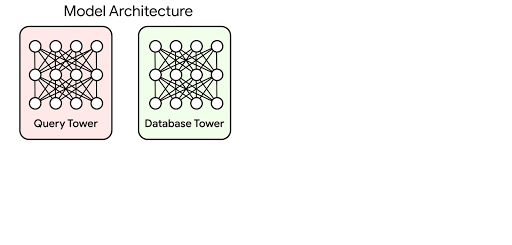### Anisotropic Vector Quantization in ScaNN

• Anisotropic vector quantization allows ScaNN to better estimate inner products that are likely to be in the top-k MIPS results and therefore achieve higher accuracy.
• On the glove-100-angular benchmark from ann-benchmarks.com, ScaNN outperformed eleven other carefully tuned vector similarity search libraries, handling roughly twice as many queries per second for a given accuracy as the next-fastest library (NGT-onng).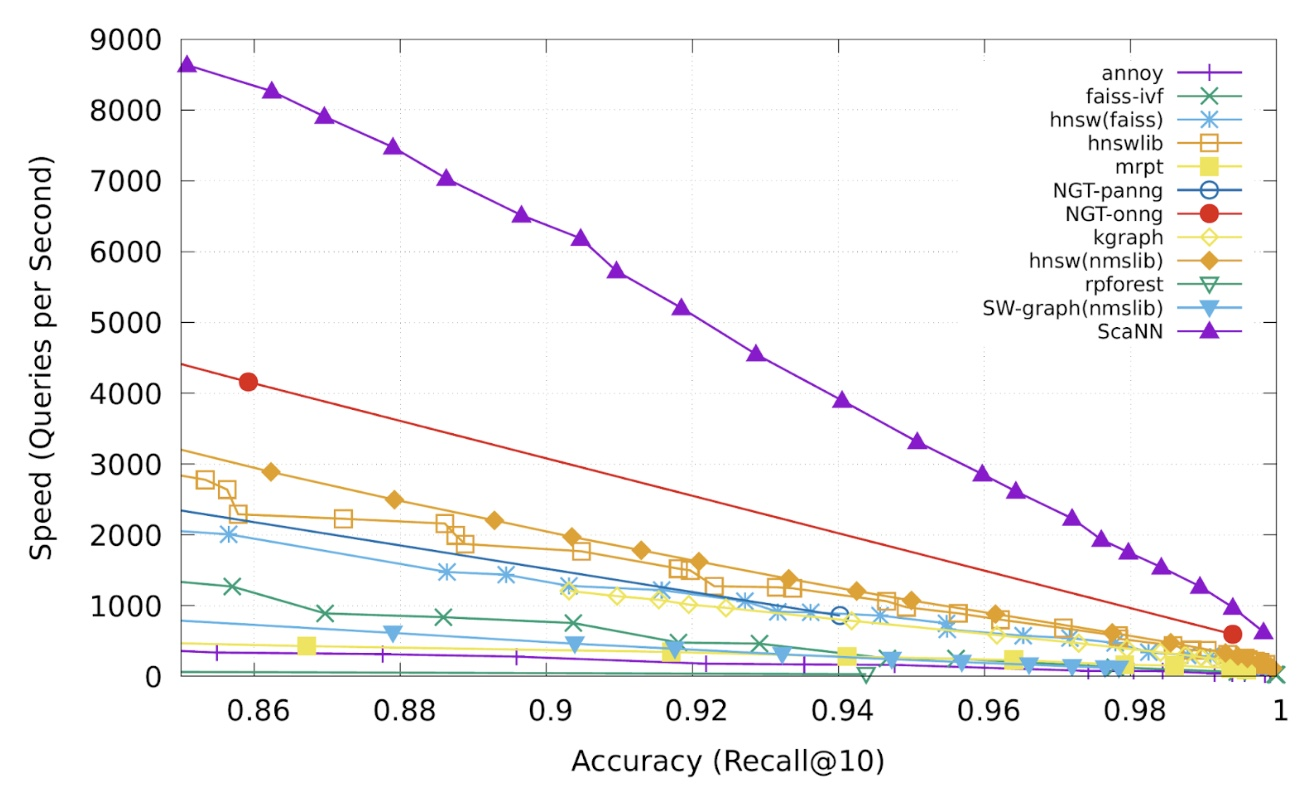• ScaNN is open-source software and you can try it yourself at GitHub. The library can be directly installed via Pip and has interfaces for both TensorFlow and Numpy inputs.

### Pros

• Handles roughly twice as many queries per second for a given accuracy as the next-fastest library.
• Anisotropic vector quantization allows ScaNN to better estimate inner products that are likely to be in the top-k MIPS results and therefore achieve higher accuracy.

### Cons

• Database size can easily be in the millions or even billions, MIPS is often the computational bottleneck to inference speed, and exhaustive search is impractical. This necessitates the use of approximate MIPS algorithms that exchange some accuracy for a significant speedup over brute-force search.

## ANNOY

• Annoy from Spotify uses a bunch of trees to enable Spotify’s music recommendations.
• In order to construct the index we create a forest (i.e., a bunch of trees). Each tree is constructed by picking two points at random and splitting the space into two by their hyperplane. We keep recursively splitting into subspaces until at most $$K$$ items are left in each node.
• It has the ability to use static files as indexes. In particular, this means you can share index across processes.
• Annoy also decouples creating indexes from loading them, so you can pass around indexes as files and map them into memory quickly.
• It tries to minimize memory footprint so the indexes are quite small.
• To understand the data structures and algorithms that Annoy uses to do approximate nearest neighbor queries, please refer Erik Bernhardsson’s Nearest neighbors and vector models – part 2 – algorithms and data structures.
• There are just two main parameters neede to tune Annoy: the number of trees n_trees and the number of nodes to inspect during searching search_k.
• n_trees is provided during build time and affects the build time and the index size. A larger value will give more accurate results, but larger indexes.
• search_k is provided in runtime and affects the search performance. A larger value will give more accurate results, but will take longer time to return.
• If search_k is not provided, it will default to n * n_trees where $$n$$ is the number of approximate nearest neighbors. Otherwise, search_k and n_trees are roughly independent, i.e. the value of n_trees will not affect search time if search_k is held constant and vice versa.
• It’s recommended to set n_trees as large as possible given the amount of memory you can afford, and it’s recommended to set search_k as large as possible given the time constraints you have for the queries.
• From Erik Bernhardsson’s Nearest neighbors and vector models – part 2 – algorithms and data structures, the diagram below shows the binary tree (left) when the subspaces have been recursively split such that there are at most $$K$$ items left in each node (right).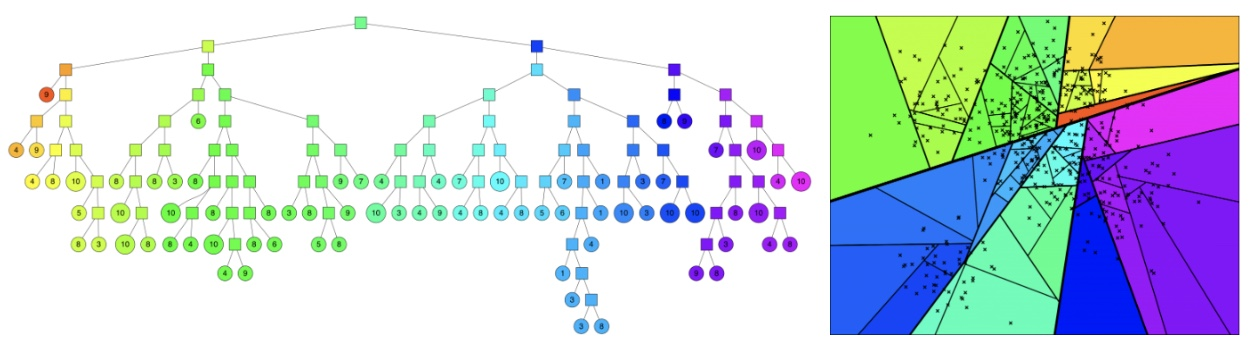• In order to search the constructed index, the forest is traversed in order to obtain a set of candidate points from which the closest to the query point is returned.

### Pros

• Decouple index creation from loading them, so you can pass around indexes as files and map them into memory quickly.
• We can tune the parameters to change the accuracy/speed tradeoff.
• It has the ability to use static files as indexes, this means you can share indexes across processes.

### Cons

• The exact nearest neighbor might be across the boundary to one of the neighboring cells.
• No support for GPU processing.
• No support for batch processing, so in order to increase throughput “further hacking is required”.
• Cant incrementally add points to it (annoy2 tries to fix this).

## Lookup/Search in Vector DBs

• Credits for this section go to Damien Benveniste.
• Vector databases are often used for recommender engines where we learn vector representations of users and items we want to recommend. This allows to quickly find similar items by using an approximate nearest neighbor search. As long as we can learn a vector representation of a piece of data, we can index it in a vector database. With the recent advent of LLMs, it became easier to compute vector representations of text documents capturing the semantic meaning of that text and vector databases make it easier to find semantically similar text documents.
• When looking for the nearest neighbors, it is often not important to be perfectly accurate. Product Quantization (PQ) is a way to quantize the vector space to represent vectors with less precision. The idea is a to cluster vectors and index the cluster centroids instead of the vectors themselves. When looking for the nearest neighbors to a query vector, we just need to pull the vectors from the closest clusters. It is a faster search and indexing the vectors takes much less memory space.
• We first need to partition each vector into smaller vectors and run a K-means algorithm on each partition. Instead of indexing the vectors, we index the centroid of the clusters they belong to. If we use 2 clusters per partition and have 6 vectors, that’s 3X data compression. Obviously, compression would be much higher with more vectors. Each vector now maps to a set of clusters and their related centroids.
• If we want to find the nearest neighbors from a query vector, we measure the squared Euclidean distance for each cluster in each partition and return the vectors with the lowest summed squared Euclidean distances.
• Instead of having to iterate through each vector, we just need to iterate through the clusters’ centroids. There is a balance between search latency and accuracy. The more clusters we use, the better will be the hash and more accurate will be the returned nearest neighbors, but it will increase the search latency as we will need to iterate through more clusters.
• This is still a brute force approach as the algorithm scales with the number of clusters but it can be used in combination with other algorithms to have blasting fast retrieval.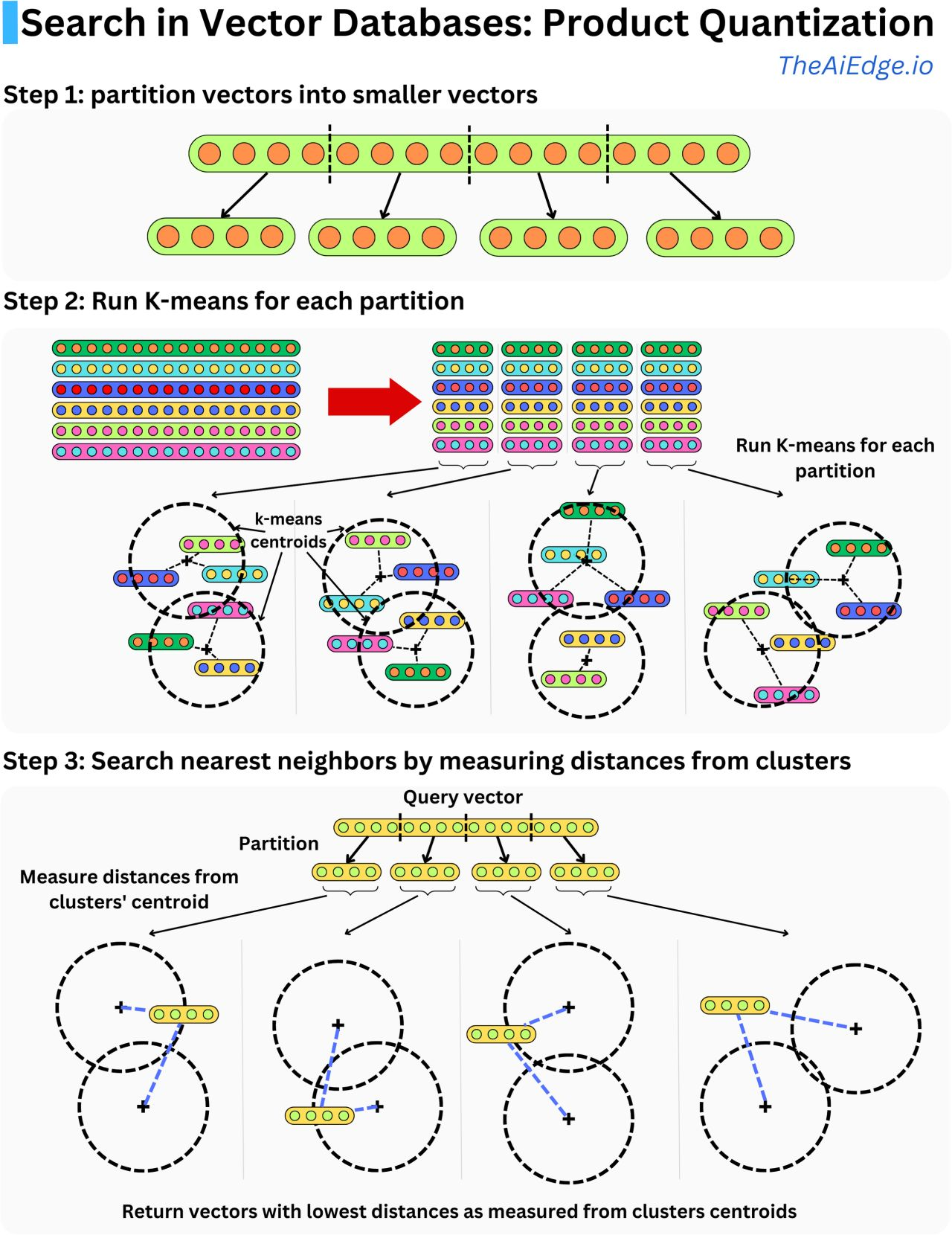## Citation

If you found our work useful, please cite it as:

@article{VatsChadha2020DistilledSimilaritySearch,
title   = {Similarity Search},
author  = {Vats, Arpita and Chadha, Aman},
journal = {Distilled AI},
year    = {2022},
note    = {\url{https://aman.ai}}
}